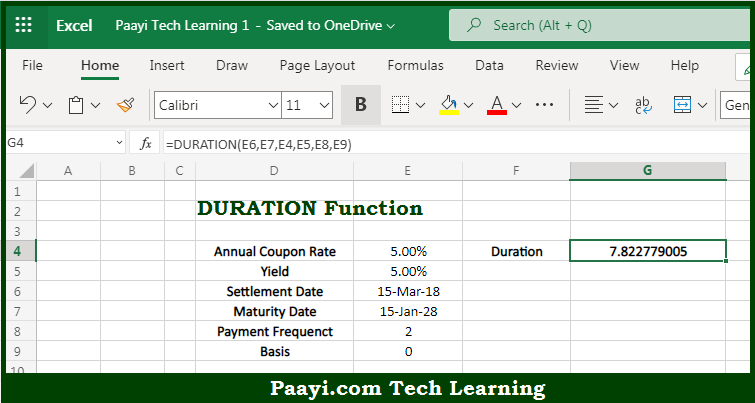# Learn How to Use Microsoft Excel DURATION Function

Written by | 0 Comments | 582 Views

In this article, you will learn how to use the Microsoft Excel DURATION function and its prime function in Microsoft Excel. You will also get to know the Microsoft Excel DURATION function return value and syntax with the help of some examples.

Microsoft Excel DURATION Function

The main purpose of the Microsoft Excel DURATION function is to get the annual duration with the periodic interest. That implies, with the help of the DURATION function you can able to return the annual duration of a security with periodic interest payments, calculated with the Macauley duration formula. So, with the help of the DURATION function, you can able to get the annual duration with the periodic interest.

Return Value of DURATION Function

The return value will be the duration in years.

Syntax of DURATION Function

=DURATION(settlement, maturity, coupon, yld, freq, [basis])

Where the arguments:

• settlement: This is the settlement date of the security.
• maturity: This is the maturity date of the security.
• coupon: This is the security's annual coupon rate.
• yld: This is the security annual's yield.
• freq: This is the number of coupon payments per year (annual = 1, semi-annual = 2, quarterly = 4).
• basis: This is the day count basis, defaults to 0 (optional).

How to Use Microsoft Excel DURATION Function?So we know that Microsoft Excel DURATION function you can able to get the annual duration with the periodic interest. That implies, with the help of the DURATION function you can able to return the annual duration of a security with periodic interest payments, calculated with the Macauley duration formula. So, with the help of the DURATION function, you can able to get the annual duration with the periodic interest.